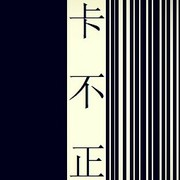[Am]一干而[G]尽 爱恨[F]嗔痴的幻[C]影 我敬[F]你 一[G]杯一干二净[C]的黎[E7]明 [Am]我在南[G]极 憧憬[F]你的北极[C]星 我等[F]你 不[G]信 心心不相[Am]印 [Am] {start_of_chorus} 你是天[Dm]意 你是[G]达达的马[C]蹄 滚滚[F]了我的红[Dm]尘 苦苦[Dm]追寻冰天[E7]雪地 [E7] {end_of_chorus} {start_of_chorus} 一寸[F]光阴一寸[G]心 一朵[Em7]昙花一朵[Am]云 一朵[F]雪花 一朵[G]梦境 一一[C]捧在手掌[Am]心 一颗[F]尘埃一菩[G]提 一颗[Em7]流星一个[Am]你 一心[F]一意捧在[G]手掌[Am]心 [Am] {end_of_chorus} [F]_ _ [G]_ _ [Em7]_ _ [Am]_ _ [Am]_ _ [Am]_ _ [Am]七世夫[G]妻 只是[F]神话的魔[C]镜 第七[F]夕 只[G]能再等一世[Am]纪 [Am] {start_of_chorus} 你是天[Dm]地 你是[G]风雨你是[C]晴 你是[F]温柔的叛[Dm]逆 逆转[Dm]我的一年[E7]四季 [E7] {end_of_chorus} {start_of_chorus} 一寸[F]光阴一寸[G]心 一朵[Em7]昙花一朵[Am]云 一朵[F]雪花 一朵[G]梦境 一一[C]捧在手掌[Am]心 一颗[F]尘埃一菩[G]提 一颗[Em7]流星一个[Am]你 一心[F]一意捧在[G]手掌[Am]心 [Am] {end_of_chorus} 偏偏[F]我越抱越[F]紧 偏我[Fm]越爱越贪[Fm]心 偏[Fm]偏要爱到[Fm]万箭穿了[G]心 [G] 才死[E7]心 [E7] {start_of_chorus} 左手[F]掌握着空[G]心 右手[Em7]掌握着痴[Am]心 十指[F]紧扣一本[G]心经 刻骨[C]铭心着苦[Am]心 可不[F]可以不甘[G]心 可不[Em7]可以不认[Am]命 如果[F]可以 拿我[G]换给[Am]你 [Am] {end_of_chorus} {title:手掌心} {artist:丁当} {author:_wc_wc_}_wc_wc_